Most Affordable JEE | NEET | 8,9,10 Preparation by Kota's Top IITian Doctor Faculties

# NCERT Solutions for Class 9 Maths chapter 13 Exercise 13.7 - Surface Areas and Volumes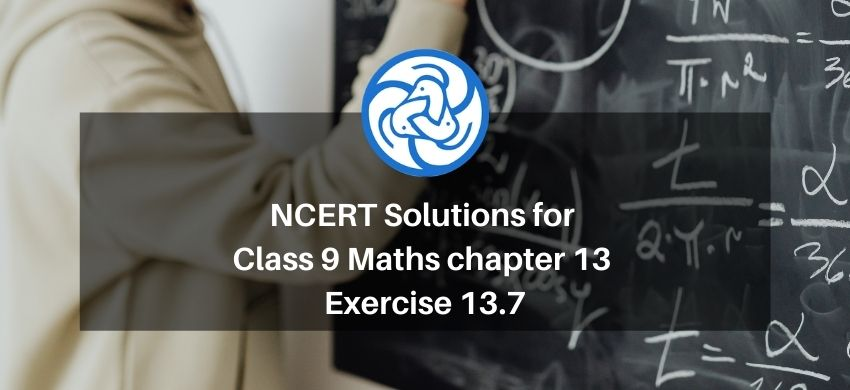Hey, are you a class 9 Student and Looking for Ways to Download NCERT Solutions for Class 9 Maths chapter 13 Exercise 13.7? If Yes then you are at the right place.

Here we have listed Class 9 maths chapter 13 exercise 13.7 solutions in PDF that is prepared by Kota’s top IITian’s Faculties by keeping Simplicity in mind.

If you want to score high in your class 9 Maths Exam then it is very important for you to have a good knowledge of all the important topics, so to learn and practice those topics you can use eSaral NCERT Solutions.

So, without wasting more time Let’s start.

### Download The PDF of NCERT Solutions for Class 9 Maths chapter 13 Exercise 13.7 "Surface Areas and Volumes"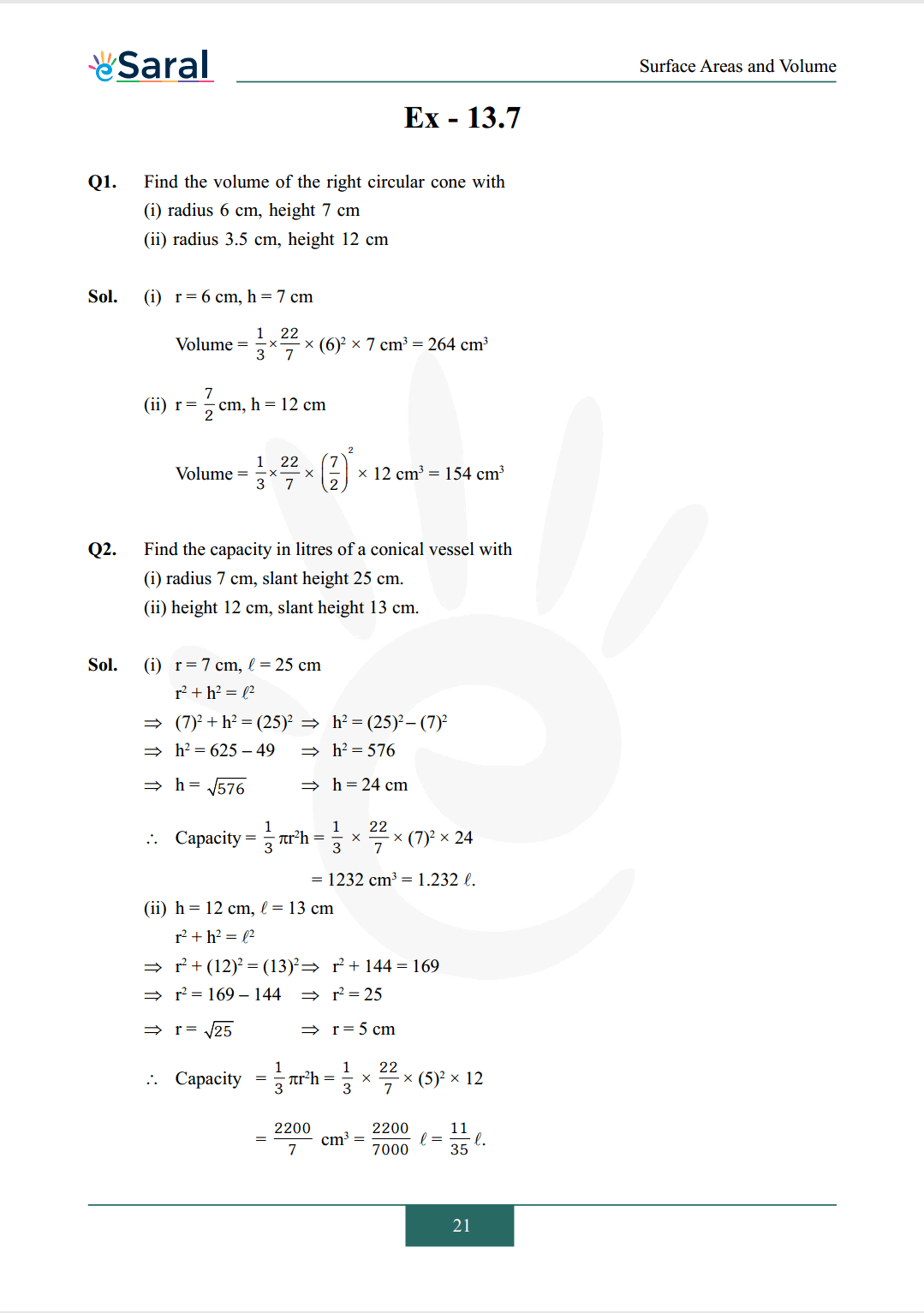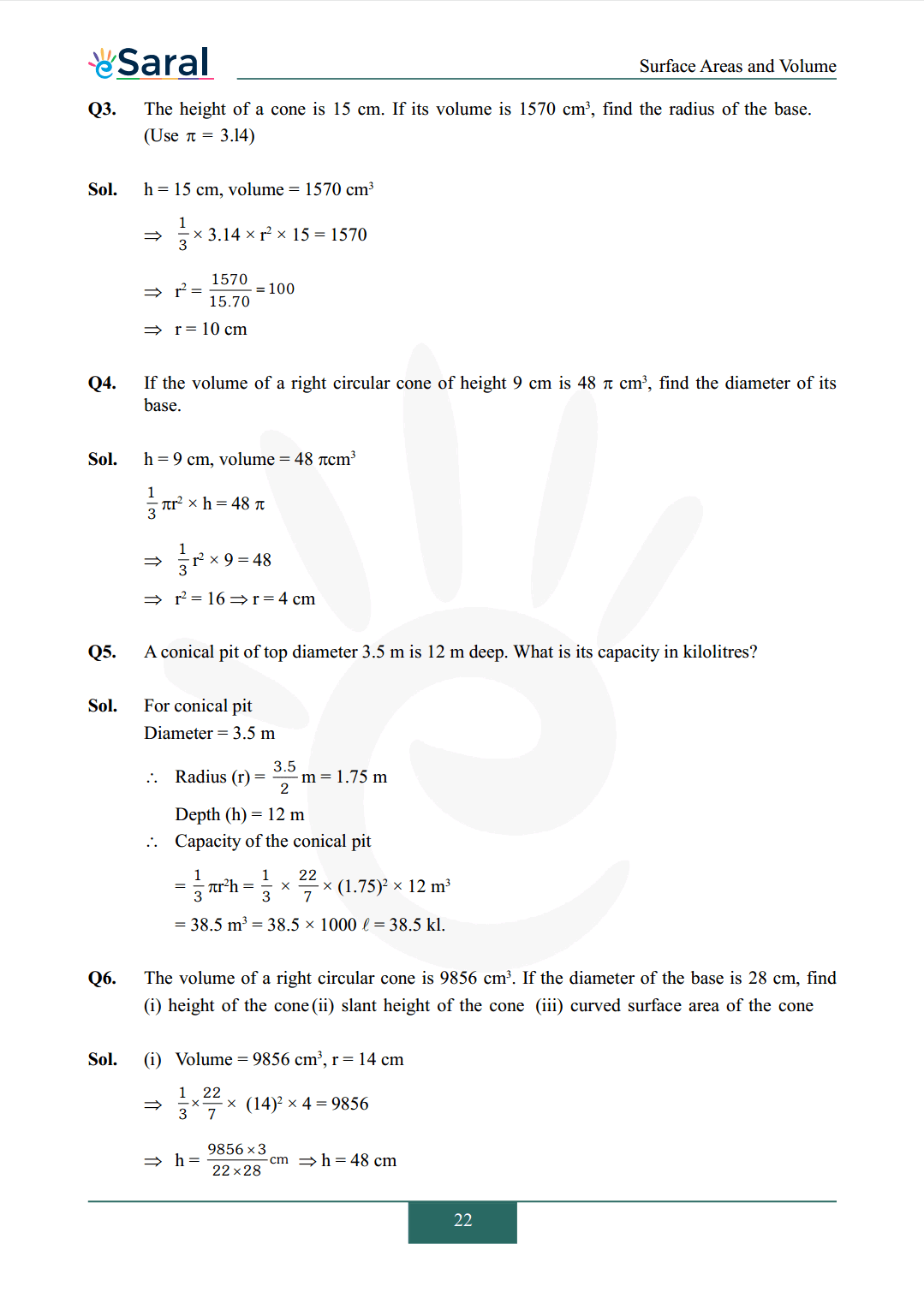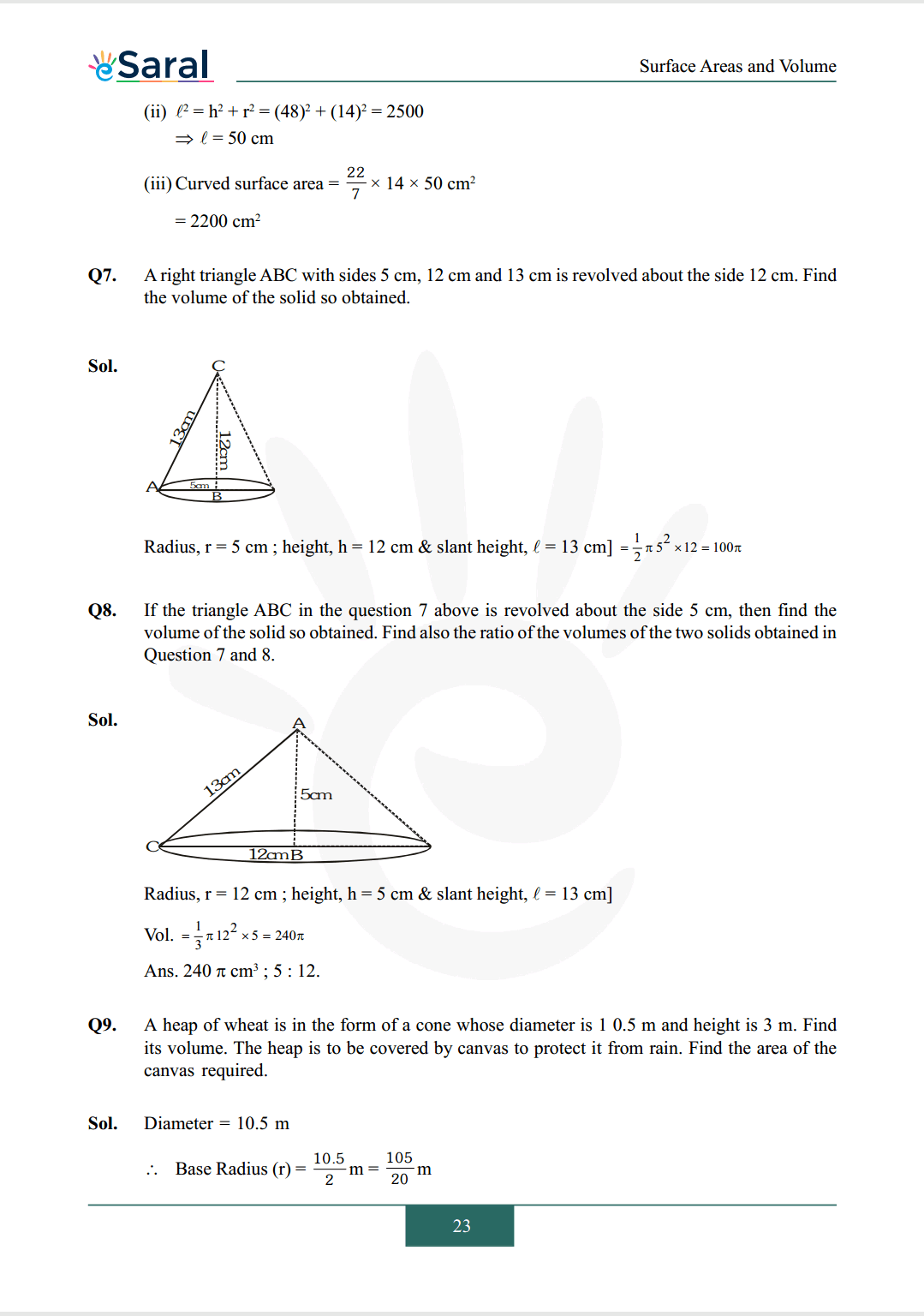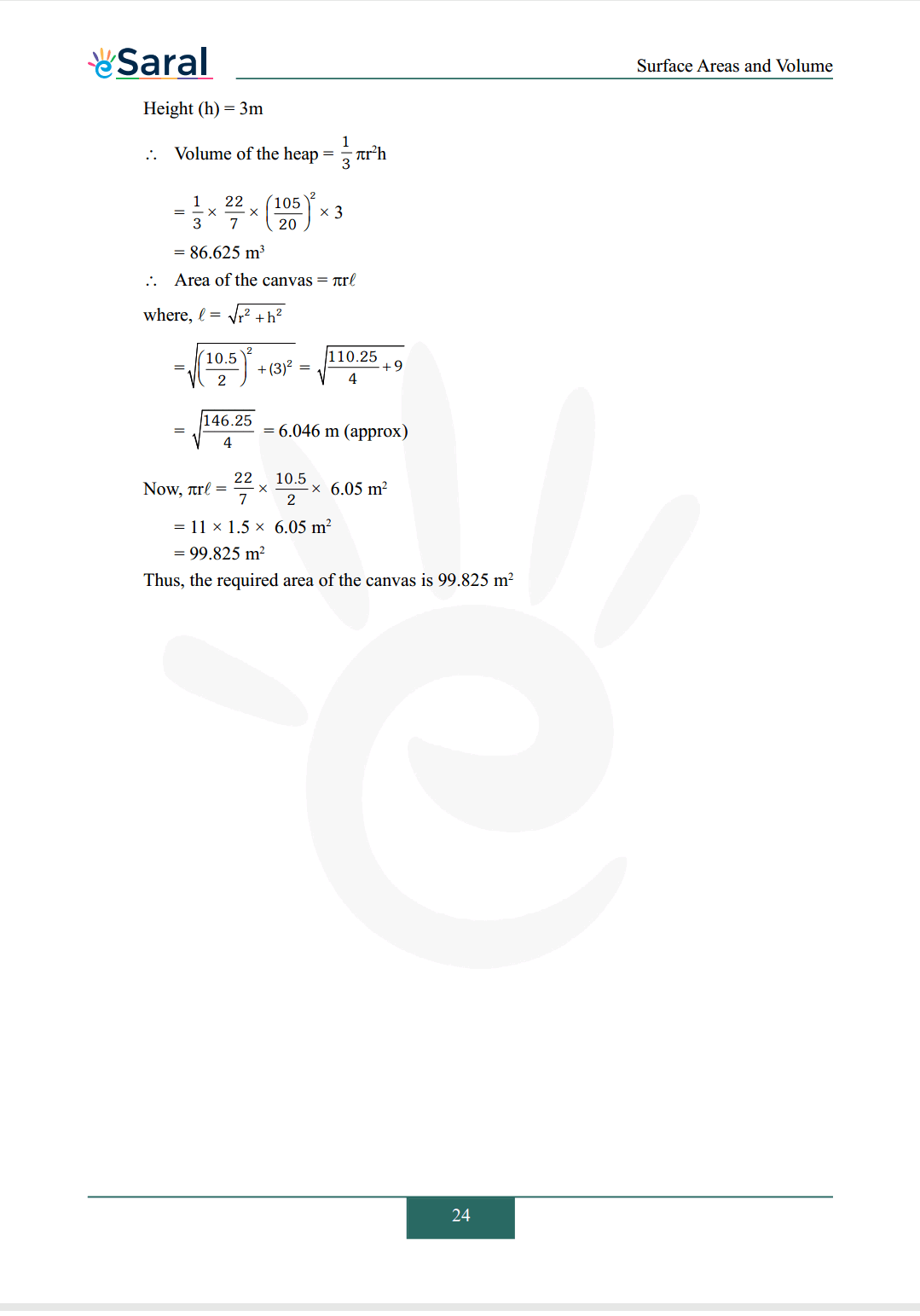#### All Questions of Chapter 13 Exercise 13.7

Once you complete the chapter 13 then you can revise Ex. 13.7 by solving following questions

Q1. Find the volume of the right circular cone with
(i) radius $6 \mathrm{~cm}$, height $7 \mathrm{~cm}$
(ii) radius $3.5 \mathrm{~cm}$, height $12 \mathrm{~cm}$

Q2. Find the capacity in litres of a conical vessel with
(i) radius $7 \mathrm{~cm}$, slant height $25 \mathrm{~cm}$.
(ii) height $12 \mathrm{~cm}$, slant height $13 \mathrm{~cm}$.

Q3. The height of a cone is $15 \mathrm{~cm}$. If its volume is $1570 \mathrm{~cm}^{3}$, find the radius of the base. (Use $\pi=3.14$)

Q4. If the volume of a right circular cone of height $9 \mathrm{~cm}$ is $48 \pi \mathrm{cm}^{3}$, find the diameter of its base.

Q5. A conical pit of top diameter $3.5 \mathrm{~m}$ is $12 \mathrm{~m}$ deep. What is its capacity in kiloliters?

Q6. The volume of a right circular cone is $9856 \mathrm{~cm}^{3}$. If the diameter of the base is $28 \mathrm{~cm}$, find
(i) height of the cone
(ii) slant height of the cone
(iii) curved surface area of the cone

Q7. A right triangle $\mathrm{ABC}$ with sides $5 \mathrm{~cm}, 12 \mathrm{~cm}$ and $13 \mathrm{~cm}$ is revolved about the side $12 \mathrm{~cm}$. Find the volume of the solid so obtained.

Q8. If the triangle $\mathrm{ABC}$ in the question 7 above is revolved about the side $5 \mathrm{~cm}$, then find the volume of the solid so obtained. Find also the ratio of the volumes of the two solids obtained in Question 7 and 8.

Q9. A heap of wheat is in the form of a cone whose diameter is $10.5 \mathrm{~m}$ and height is $3 \mathrm{~m}$. Find its volume. The heap is to be covered by canvas to protect it from rain. Find the area of the canvas required.